Reach Us+44-7482-875032
The Inverse Derivative - The New Algorithm of the Derivative | OMICS International
Journal of Applied & Computational Mathematics
All submissions of the EM system will be redirected to Online Manuscript Submission System. Authors are requested to submit articles directly to Online Manuscript Submission System of respective journal.

# The Inverse Derivative - The New Algorithm of the Derivative

GuagSan Y*

Harbin Macro Dynamics Institute, P. R. China.

*Corresponding Author:
GuagSan Y
Harbin Macro Dynamics Institute
150066, P. R. China
Tel: +8645186412847
E-mail: [email protected]

Received Date: April 18, 2016; Accepted Date: June 24, 2016; Published Date: June 30, 2016

Citation: GuagSan Y (2016) The Inverse Derivative - The New Algorithm of the Derivative. J Appl Computat Math 5: 311. doi:10.4172/2168-9679.1000311

Copyright: © 2016 GuagSan Y. This is an open-access article distributed under the terms of the Creative Commons Attribution License, which permits unrestricted use, distribution, and reproduction in any medium, provided the original author and source are credited.

Visit for more related articles at Journal of Applied & Computational Mathematics

#### Abstract

The Newton’s second law and third law has proven to be wrong, his mistake is the derivative operation the limitation resulting. Therefore new derivative the computation method desiderate the creation, this is the Inverse Derivative algorithm. The Inverse Derivative is a kind of extension of the derivative computation, it is based on the derivative calculated generated, using most of the derivative formulas to converted adjusted. In the field of physics and mechanical engineering, Inverse Derivative may be a large number of employed, to replace original derivative computation method. Will produce the enormous and profound influence.

#### Keywords

Inverse derivative; Contrary derivative; Inverse differential; Contrary differential; Inverse integral

#### Introduction

This paper is in a basis of the derivative, bring forward a new calculation method-Inverse Derivative.

#### The Inverse Derivative Principle

For functions:

y=f(x)                                         (1)

In this case the derivative is: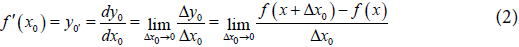It shows that when the increment Δx0 approaches zero, the limit of Δy0x0 namely the y respect to x the derivatives. Here make incremental Δx0 approaches zero, which is very important. It shows the derivative f '(x0) is a unit of differential dx0 to calculated. For example, when the derivative is represented with speed, it is in units of time, That is in time of unit, by changing the distance traveled, to represent the rate of change of speed.

About Inverse Derivative, it is different from the derivative of it? First, look at the inverse function:

x=g(y)                                       (3)

Or:

x=f-1(y)                                   (4)

Well, that is the Inverse Derivative: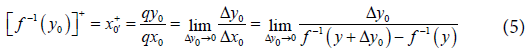So the Inverse Derivative is recorded as [f-1(y0)]+,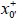, and qy0/qx0. Corresponds with the differential of derivative qy0 and qx0 also called Inverse Differential [1-5].

Clearly, in the Inverse Derivative operations, is when incremental Δy0 approaches zero, for Δy0x0 seek limit. Therefore, the Inverse Derivative [f-1(y0)]+ to indicate is with Inverse Differential qy0 for the units to calculated. Here it with the derivative calculate, been have essentially different. For example, when by the Inverse Derivative represented speed, it is with move distance for unit. That is within the distance of units, through experienced time its different, and represent the speeds is different. This is with the derivative to record or described speed, is had significantly different. And it versus the thing the rule of physics, and even there will be different interpretations. So this is very important.

In the course of physics, many calculations should be use the Inverse Derivative to calculate, it will be correct. For example, concerning force the calculate, decided size of the force, moving objects the speed or acceleration, is by the object the displacement, to direct reflection the energy of the movement of objects. In this time denote the object move the velocity and acceleration, its at move the unit space by experienced the time, reflect then is the object locomotion energy to relatively to the density of the time. So the force to calculate, regardless is a velocity or acceleration, all should regard that object move space to be unit, been proceed the computation. Adopt the Inverse Derivative algorithm namely [6,7].

According to this principle, we discovered the Newton second law of the adoption derivative computation is wrong. And put forward to regard Inverse Derivative computation as fundamental, new that second laws of motion and new the third laws of motion.

Obviously, the principle of the Inverse Derivative be near to derivative very, been a derivative principle to expand. The Inverse Derivative quote the inverse function of the derivative, so it as if the reciprocal of the derivative operation. But increment Δy0 and increment Δx0 that the position, does not change however in Inverse Derivative. This is for the sake of the habit of the traditional physics in care concept, therefore just was changed by denominator the unit of the computation to numerator at this time, but denominator numerator of however the character did not change. For example while computing velocity or accelerations, is still the divide of distance of the move with undergo that the time.

So the analytic formula of the Inverse Derivative is equals to will the analytic formula inside of the derivative, after exchange of the x of the variable y will the numerator denominator exchange. And will function symbol changes to inverse function symbol.

#### The Conversion of the Derivative Formula

Because the principle of the Inverse Derivative is that principle to base on the derivative, so usually be applicable to the derivative the circumstance, also will be applicable to the Inverse Derivative equally. For example concerning whether function can seek the derivative, the Inverse Derivative is opposite with derivative. When the image of function its shows whether can seek the derivative, well then at this image of function revolving 90°, its whether can seek the Inverse Derivative to is same with it.

It is obvious, the Inverse Derivative analytic formula just will the derivative analytic formula, the numerator denominator makes exchange. Therefore, from the analytic formula of the derivative, can will derivative and differential the formula, conversion through simple adjustment to Inverse Derivative and Inverse Differential the formula .

For example the function that the sum difference that the derivative formula: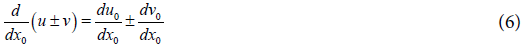and

(u ± v) = uv '                                 (7)

Can to convert the sum difference that the Inverse Derivative formula: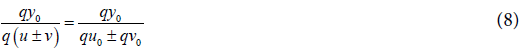and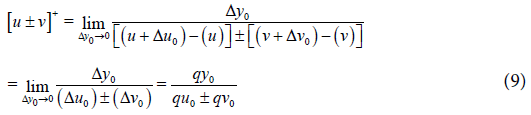And the product the derivative formula: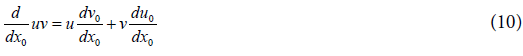and

(uv)=uv '+vu '                                 (11)

Also can convert to the Inverse Derivative formula of the product: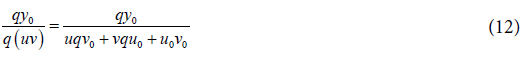and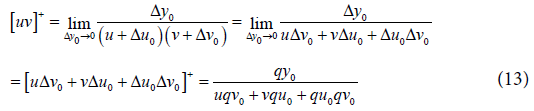And the derivative formula of the quotient: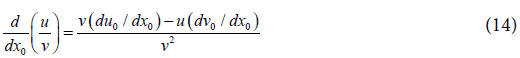and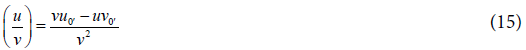Conversion to the Inverse Derivative formula is then: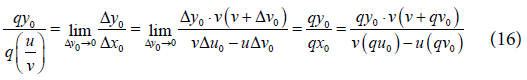and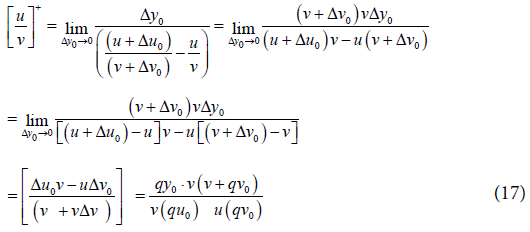The derivative formula of the composite function: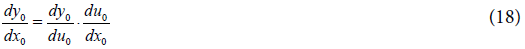and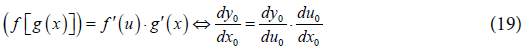Can convert directly to the formula of the Inverse Derivative: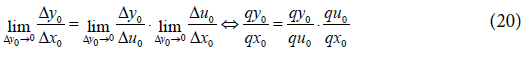and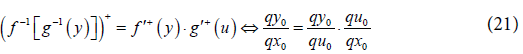The formula of the derivative of higher order(second-order):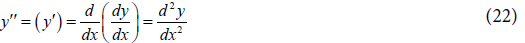Conversion the higher order(second-order) Inverse Derivative formula is then: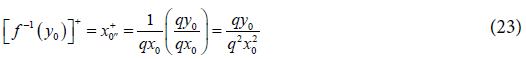Above is that some in common use derivative formula, conversion to Inverse Derivative formula the method. In need can will more derivative principle, be to conversion and quote for that the Inverse Derivative.

#### The Inverse Derivative and the Partial Derivative the Conversion

The Inverse Derivative expressive, in reality can with the partial derivative to achieve. Therefore, the Inverse Derivative can convert to the partial derivative. Whereas, the partial derivative can also convert to the Inverse Derivative [9,10].

For example the function of second variables:

z=f(x,y)                              (24)

To the y partial derivative is: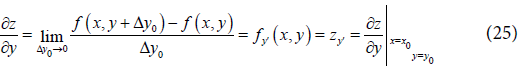Be to partial differential at this time: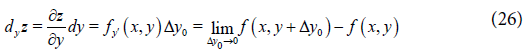Then the Inverse Derivative is: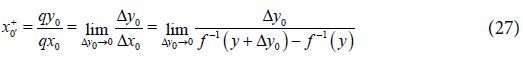Can treat Inverse Derivative as a function of second variables its obviously: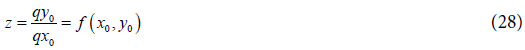Usher in to be partial differential will this function of second variables: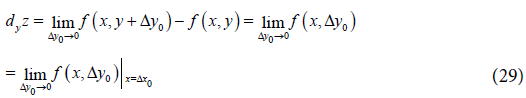Then the Inverse Derivative be for a function of second variables, been it an partial differential. Therefore it can convert to partial differential of a partial derivative, some similar condition that the partial differential of partial derivative, also can convert to the Inverse Derivative.

#### The Applied of the Inverse Derivative - New Second Motion Laws and Third Motion Laws

The typical applied of the Inverse Derivative, it is in new second motion laws and third motion laws.

New the second laws of motion: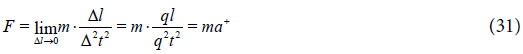It too with mass and the product representation force of the acceleration, but its acceleration is second-order Inverse Derivative, record for a+ to express it is different with that the derivative. Because at this time second-order the Inverse Differential q2t2, just is among them the variable by the Inverse Derivative. So when the force changes, among them mass m with force is relation to geometric proportion, but its acceleration a+ then is relation to an inverse proportion square with force.

This will be caused a series of singular result, express for the same force, when the mass of the object is different, the mass of the force is with the value of the product of the acceleration is different, it will is. Theory deduce with experiment all denote, this is exactness is it. But Newton the second laws of motion and the third laws of motion, because is an adoption derivative operation, therefore in reality is wrong.

New the Inverse Derivative formula of the third laws of motion: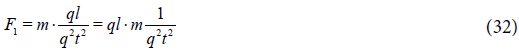and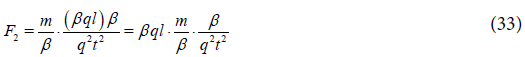It is explained two objects interactional, the small object in mass suffers the larger dynamic acting force to action. Then when two mass is different the object interactional, two objects produces the different momentum, therefore its momentum is not conservation. New the third laws of motion expresses, the momentum is not conservation, for this reason classical mechanics inside, the momentum conservation law also is wrong.

The above circumstance indicate, Inverse Derivative is in the physics and the mechanics to used, produce the strange result. There is evidence showing, Inverse Derivative is in electrodynamics or electromagnetics realms, also may have the very big use .

#### Inverse Integral

The integral of the Inverse Derivative computes, being called the Inverse Integral. It computes with common integral, should has no too big differentiate. It is will the common integral the compute that the differential symbol, changing the symbol of the inverse differential is then. The principle and method of its computation is similar is it. But although the superficial computing method is same, and because of the character of the Inverse Derivative, the result that computation come out will still have very big different.

For example compute the force to does work is it: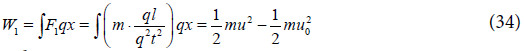and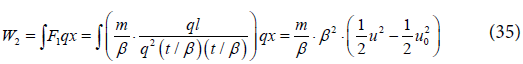Express the same force action to the different object of two masses, the force to two the object those make the work is different. The object of small β times of the mass, by the force that big β times of make the work. This a kind circumstance gained the proof in of experiment of elasticity release of spring. This point also is very important. So operation in above integral and the experiment of the spring all expresses, the spring elasticity releases, to masses different the object, engender the different work. This a circumstance is shows, when the spring confront the different object in masses does the work, the energy is not conservation.

For this reason Inverse Derivative and the adduction of the Inverse Integral computation, cause some important physics law been by shaken. Among them of the meaning is enormous.

#### Conclusion

Therefore by complement and expand of the Inverse Derivative for derivative operation, is extremely important. In actuality adopt similar Inverse Derivative such method, to the thing come proceed measure or formulations, is also do not lack its example in fact. For example, the running that athletics compete the inside, is all in measure rule the distance, that consume the time. And is not in restrict time, run how much distances. For example in physics, usually the problem that discuss is, certain energy the action in stated the time. For example the force, electromagnetism force, electric quantity and so forth. This paper inside mentions, is the classical mechanics the second third laws of motion, and new the second third laws of motion that the force. Because of the adoption of the Inverse Derivative, the law of the some physics was wrong by the proof. That very much is may that the similar circumstance, will expand to include the other one physics course of electromagnetics and so on. And creation important influence.

Thereby discover and put forward this kind of new computing method of the Inverse Derivative, the meaning is extremely important. To the development and progress of human science, inevitable creation profound and extensive influence.

#### Acknowledgement

The author thank his professors, for their support (Professor Shixu Guan, Chief editor Xinmin Zhu, Principal Lanxu Xu). The author also thank his the university for assistance (Department head Shuquan Wang, Department head Xinde Jiang, Associate Professor Risheng Piao, and many teachers).

#### References

Select your language of interest to view the total content in your interested language

### Article Usage

• Total views: 9674
• [From(publication date):
June-2016 - Aug 26, 2019]
• Breakdown by view type
• HTML page views : 9555
• PDF downloads : 119

## Post your commentCan't read the image? click here to refresh
###### Peer Reviewed Journals

Make the best use of Scientific Research and information from our 700 + peer reviewed, Open Access Journals

International Conferences 2019-20

Meet Inspiring Speakers and Experts at our 3000+ Global Annual Meetings

Top